# 张量网络算法基础（八、张量网络机器学习【上】）

### 张量网络机器学习# 一、机器学习基本思想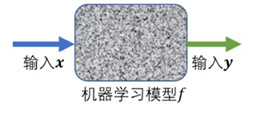$f={\sigma }_{2}\left(\stackrel{^}{W}{\sigma }_{1}\left(\stackrel{^}{W}x+{b}_{1}\right)+{b}_{2}\right)$f=σ2​(W^σ1​(W^x+b1​)+b2​)# 二、希尔伯特空间、特征映射与量子概率$\mid {x}_{l}^{\left[n\right]}⟩=\mathrm{cos}\frac{{x}_{l}^{\left[n\right]}\pi }{2}\mid 0⟩+\mathrm{sin}\frac{{x}_{l}^{\left[n\right]}\pi }{2}\mid 1⟩$∣∣∣​xl[n]​⟩=cos2xl[n]​π​∣0⟩+sin2xl[n]​π​∣1⟩

$\mid {S}^{\left[n\right]}⟩=\prod _{\otimes l=1}^{L}\mid {{x}_{i}}^{\left[n\right]}⟩$∣∣∣​S[n]⟩=⊗l=1∏L​∣∣∣​xi​[n]⟩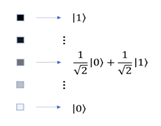$P\left({y}_{1},\dots ,{y}_{L}\right)=\left(\prod _{\otimes l=1}^{L}⟨{y}_{l}\mathrm{\mid }\psi ⟩\right)$P(y1​,…,yL​)=(⊗l=1∏L​⟨yl​∣ψ⟩)

$\sum _{{y}_{1},\dots ,{y}_{L}=1}^{D}\prod _{\otimes l=1}^{L}\mid {y}_{l}⟩⟨{y}_{l}\mid =I$y1​,…,yL​=1∑D​⊗l=1∏L​∣yl​⟩⟨yl​∣=I

$\sum _{{y}_{1},\dots ,{y}_{L}=1}^{D}\text{P}\left({y}_{1},\dots ,{y}_{L}\right)=\sum _{{y}_{1},\dots ,{y}_{L}=1}^{D}\prod _{\otimes l=1}^{L}\mid ⟨\psi \mid {y}_{l}⟩⟨{y}_{l}\mid \psi ⟩\mid =\prod _{\otimes l=1}^{L}\mathrm{\mid }⟨\psi \mid \psi ⟩\mathrm{\mid }=1$y1​,…,yL​=1∑D​P(y1​,…,yL​)=y1​,…,yL​=1∑D​⊗l=1∏L​∣⟨ψ∣yl​⟩⟨yl​∣ψ⟩∣=⊗l=1∏L​∣⟨ψ∣ψ⟩∣=1

$\left\{{x}_{l}\right\}=\left\{{x}_{m}^{\left[A\right]}\right\}\cup \left\{{x}_{n}^{\left[\text{B}\right]}\right\}${xl​}={xm[A]​}∪{xn[B]​}

${\stackrel{^}{\rho }}^{\left[B\right]}={\mathrm{Tr}}_{}\mathrm{\mid }\phi ⟩⟨\phi \mathrm{\mid }$ρ^​[B]=Tr{xm[A]​}​∣φ⟩⟨φ∣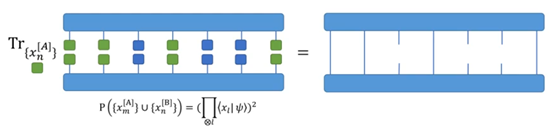$\text{P}\left(\left\{{x}_{n}^{\left[\text{B}\right]}\right\}\right)=\prod _{\otimes n}⟨{x}_{n}^{\left[\text{B}\right]}\mid {\stackrel{^}{\rho }}^{\left[B\right]}\mid {x}_{n}^{\left[\text{B}\right]}⟩$P({xn[B]​})=⊗n∏​⟨xn[B]​∣∣∣​ρ^​[B]∣∣∣​xn[B]​⟩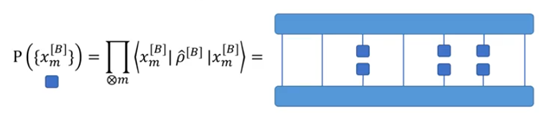# 三、等概率先验假设与量子懒惰学习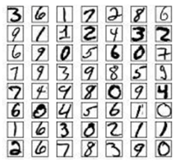$\mathrm{P}\left(\mathbit{X}\right)={\left(\prod _{\otimes l=1}^{L}\mid ⟨{x}_{l}\mid \psi ⟩\mid \right)}^{2}=\text{const.}\mathrm{\forall }\mathbf{X}\propto \mathrm{x}$P(X)=(⊗l=1∏L​∣⟨xl​∣ψ⟩∣)2=const.∀X∝x

$\mid {\psi }^{\text{lazy}}⟩=\frac{1}{\sqrt{\mathrm{\mid }\text{x}\mathrm{\mid }}}\sum _{X\propto \text{x}}\prod _{\otimes l=1}^{L}\mid {x}_{l}⟩$∣∣​ψlazy⟩=∣x∣​1​X∝x∑​⊗l=1∏L​∣xl​⟩

$⟨{\psi }^{\text{lazy}}\mid {\psi }^{\text{lazy}}⟩=\frac{1}{\mathrm{\mid }x\mathrm{\mid }}\sum _{X,{X}^{\mathrm{\prime }}{\propto }_{X}}⟨X\mid {X}^{\mathrm{\prime }}⟩\approx \frac{1}{\mathrm{\mid }\mathbb{X}\mathrm{\mid }}\sum _{X,{X}^{\mathrm{\prime }}{\propto }_{X}}{\delta }_{X,{X}^{\mathrm{\prime }}}=1$⟨ψlazy∣ψlazy⟩=∣x∣1​X,X′∝X​∑​⟨X∣X′⟩≈∣X∣1​X,X′∝X​∑​δX,X′​=1

$\mid {\psi }_{k}^{\text{lazy}}⟩=\frac{1}{\sqrt{\mid {\text{x}}_{k}\mid }}\sum _{\text{X}\propto {\text{x}}_{k}}\prod _{\otimes l=1}^{L}\mid {x}_{l}⟩$∣∣∣​ψklazy​⟩=∣xk​∣​1​X∝xk​∑​⊗l=1∏L​∣xl​⟩

${P}_{k}\left(\mathbf{Y}\right)=\mathrm{\mid }⟨\mathbf{Y}\mid {\psi }_{k}^{\text{lazy}}⟩{\mathrm{\mid }}^{2}$Pk​(Y)=∣⟨Y∣ψklazy​⟩∣2